# 날짜 참조 테이블 만들기

2017.01.11 08:36 Views : 3166

날짜 참조 테이블 만들기

• Version : SQL Server 2005, 2008, 2008R2, 2012, 2014

데이터를 검색 할 때 가장 많이 사용되는 조건 중 하나가 날짜 일 것이다. 다양한 통계 쿼리를 만들다 보면 날짜 범위에 관한 조건문이 많이 사용되는데 이때 해당 기간을 검색하기 위한 참조 테이블을 만들어 사용하면 편리하다. (흔히 날짜 차원 테이블이라고 부른다.)

날짜 참조 테이블을 생성한다.

 USE TEMPDB  IF OBJECT_ID('dbo.#t') is not null DROP TABLE dbo.#t; CREATE TABLE #t ( [Date] datetime , [Year] smallint , [Quarter] tinyint , [Month] tinyint , [Day] smallint -- from 1 to 366 = 1st to 366th day in a year , [Week] tinyint -- from 1 to 54 = the 1st to 54th week in a year; , [Monthly_week] tinyint -- 1/2/3/4/5=1st/2nd/3rd/4th/5th week in a month , [Week_day] tinyint -- 1=Mon, 2=Tue, 3=Wed, 4=Thu, 5=Fri, 6=Sat, 7=Sun ); GO

날짜 정보를 생성 한다. 아래 스크립트의 경우 Week 컬럼의 시작 요일은 월요일이다. 시작 요일을 일요일로 바꾸고 싶으면 -1을 한다.

 -- populate the table D_Date, and the day of week is defined as -- 1=Mon, 2=Tue, 3=Wed, 4=Thu,5=Fri, 6=Sat, 7=Sun ;WITH C0 AS (SELECT c FROM (VALUES(1),(1)) AS D(c)), C1 AS (SELECT 1 AS c FROM C0 AS A CROSS JOIN C0 AS B), C2 AS (SELECT 1 AS c FROM C1 AS A CROSS JOIN C1 AS B), C3 AS (SELECT 1 AS c FROM C2 AS A CROSS JOIN C2 AS B), C4 AS (SELECT 1 AS c FROM C3 AS A CROSS JOIN C3 AS B), C5 AS (SELECT 1 AS c FROM C4 AS A CROSS JOIN C3 AS B), C6 AS (select rn=row_number() over (order by c) from C5), C7 as (select [date]=dateadd(day, rn-1, '19000101') FROM C6 WHERE rn <= datediff(day, '19000101', '99991231')+1) INSERT INTO #t ([year], [quarter], [month], [week], [day], [monthly_week], [week_day], [date]) SELECT datepart(yy, [DATE]), datepart(qq, [date]), datepart(mm, [date]), datepart(wk, [date]) , datediff(day, dateadd(year, datediff(year, 0, [date]), 0), [date])+1 , datepart(week, [date]) -datepart(week, dateadd(month, datediff(month, 0, [date]) , 0))+1 , CASE WHEN datepart(dw, [date])+@@datefirst-1 > 7 THEN (datepart(dw, [date])+@@datefirst-1)%7 ELSE datepart(dw, [date])+@@datefirst-1 END , [date] FROM C7 --where [date] between '19900101' and '20990101'; -- if you want to populate a range of dates GO

날짜 정보가 1900-01-01부터 9999-12-31일 까지 데이터가 생성되었다. (자그마치 2958464개의 행이다.)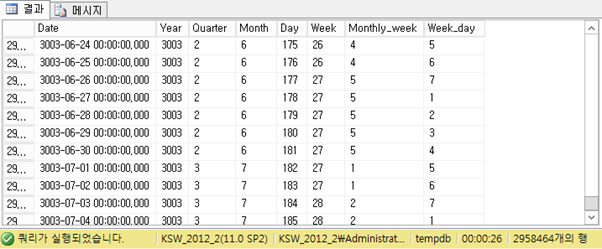[월별 첫째 날짜와 마지막 날짜 찾기]

 -- find first day of prev/curr/next month with current date declare @curr_dt datetime = '2015-02-11';  select distinct First_Mth_Day=first_value([Date]) over (partition by [month] order by [Date] asc) , Last_Mth_Day=last_value([Date]) over (partition by [month] order by [Date] asc ROWS BETWEEN Current Row AND UNBOUNDED FOLLOWING ) from #t where datediff(month, @curr_dt, [Date]) between -1 and 1 and datediff(year, @curr_dt, [Date]) between -1 and 1 order by 1 go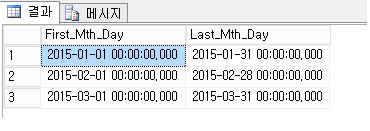[토, 일요일을 제외한 첫 날짜와 마지막 날짜 구하기]

 -- find the first/last weekday of prev/curr/next month declare @curr_dt datetime = '2015-02-11'; select distinct First_Week_Day=first_value([Date]) over (partition by [month] order by [Date] asc) , Last_Week_Day=last_value([Date]) over (partition by [month] order by [Date] asc ROWS BETWEEN Current Row AND 31 FOLLOWING ) from #t where datediff(month, @curr_dt, [Date]) between -1 and 1 and datediff(year, @curr_dt, [Date]) between -1 and 1 and Week_Day between 1 and 5 order by 1 go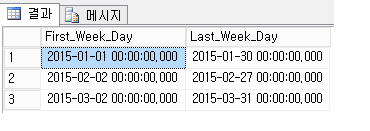[주말(토요일 또는 일요일)의 첫 날짜와 마지막 날짜 구하기]

 -- find the first / last weekend day of prev/curr/next month declare @curr_dt datetime = '2015-02-11'; with c as ( select distinct First_Wknd_Day=first_value([Date]) over (partition by [month] order by [Date] asc) , Last_Wknd_Day=last_value([Date]) over (partition by [month] order by [Date] asc ROWS BETWEEN Current Row AND UNBOUNDED FOLLOWING ) from #t where datediff(month, @curr_dt, [Date]) between -1 and 1 and datediff(year, @curr_dt, [Date]) between -1 and 1 and Week_Day between 6 and 7 ) select [Month]=choose((row_number() over (order by First_wknd_day asc)), 'Prev_Mth', 'Curr_Mth', 'Next_Mth') , First_Wknd_Day, Last_Wknd_day from c go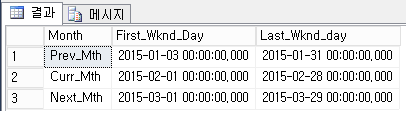[매월 주 시작 날짜와 주 마지막 날짜 구하기]

 -- find the first/last weekday in each month of all years select distinct [Year], [Month], First_Week_Day=first_value([Date]) over (partition by [year], [month] order by [Date] asc) , Last_Week_Day=last_value([Date]) over (partition by [year], [month] order by [Date] asc ROWS BETWEEN Current Row AND 32 FOLLOWING ) from #t where Week_Day between 1 and 5 -- for weekend, change to: between 6 and 7 order by 1, 2 go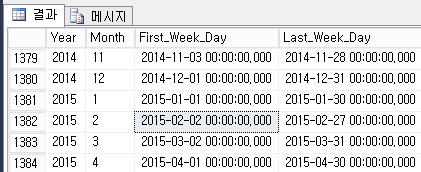[검색 기간동안 토,일요일이 몇번있는지 구하기]

 -- how many weekend days or weekdays between two dates declare @start_day datetime ='2015-01-11', @End_day datetime ='2015-02-03' select [Start_Date] = @Start_day, [End_Date]=@End_day, Total_weekend_days = count(*) from #t where (Week_Day between 6 and 7) -- for weekdays, use "between 1 and 5" and ([Date] between @start_day and @end_day) go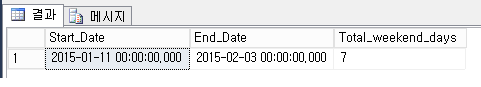[매월 2번째 주 시작날짜 구하기]

 -- find nth week/weekend day of each prev/curr/next month -- eg. find the 2nd Monday of each prev/curr/next month declare @curr_dt datetime = getdate(); ; with c as (select rn=RANK() over (partition by [month] order by [date] ASC), [Date] -- attention to 'ASC' from #t where datediff(month, @curr_dt, [Date]) between -1 and 1 and datediff(year, @curr_dt, [Date]) between -1 and 1 and Week_Day = 1 -- 1=Mon, 2=Tue,... 7=Sun ) select [Month]=choose((row_number() over (order by (select null))), 'Prev_Mth', 'Curr_Mth', 'Next_Mth') ,[2nd_Monday] = [Date] from c where rn=2 -- nth, for example if finding the 3rd Monday, set rn=3 order by 2 go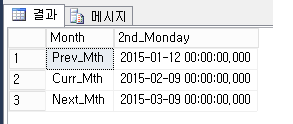[매월 마지막 2번쨰 주 마지막 날짜 구하기]

 -- find the nth last week/weekend day of prve/curr/next month -- eg. find the 2nd last Sat of prev/curr/next month declare @curr_dt datetime = getdate(); ; with c as (select rn=RANK() over (partition by [month] order by [date] DESC), [Date] -- attention to 'DESC' from #t where datediff(month, @curr_dt, [Date]) between -1 and 1 and datediff(year, @curr_dt, [Date]) between -1 and 1 and Week_Day = 6 -- 1=Mon, 2=Tus, ... 7=Sun ) select [Month]=choose((row_number() over (order by (select null))), 'Prev_Mth', 'Curr_Mth', 'Next_Mth') , [2nd_Last_Sat]=[Date] from c where rn=2 order by 2 go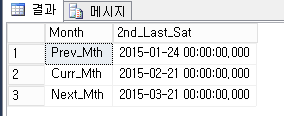[참고자료]

강성욱 / jevida@naver.com
Microsoft SQL Server MVP
Blog : http://sqlmvp.kr

No. Subject Author Date Views
Notice 2023년 1월 - SQLER의 업데이트 강좌 리스트 2023.01.02 1249
2006 인덱스 유지관리 작업과 SQL Server 쿼리 성능 2017.01.11 3399
2005 네트워크 드라이브에 데이터베이스 복원하기 2017.01.11 4231
2004 확장 저장 프로시저를 활용한 논리디스크 용량 확인 2017.01.11 2508
» 날짜 참조 테이블 만들기 2017.01.11 3166
2002 인덱스 상세 정보 확인 2017.01.11 3820
2001 DTC Transacntion 오버헤드 2017.01.11 1319
2000 대용량 로드를 위한 BULK INSERT 옵션 2017.01.11 5492
1999 SQL Server 2014 Diagnostic Information Queries 2017.01.11 1644
1998 SQL Server 2012 Contained Database 2017.01.11 1047
1997 SQL Server 2008R2 Diagnostic Information Queries 2017.01.11 1447
1996 SQL Server 2005 Diagnostic Information Queries 2017.01.11 1387
1995 601 Error, Could not continue scan with NOLOCK due to SQL Server data Movement 2017.01.11 3959
1994 데이터베이스의 모든 인덱스 생성 삭제 스크립트 만들기 2017.01.11 1702
1993 SQL Server Spinlock 소개 2017.01.11 1620
1992 Ad-hoc 쿼리와 실행계획 2017.01.11 2613
1991 로그인 계정이 접근할 수 있는 데이터베이스 확인 2017.01.11 3080
1990 클러스터된 SQL 서버 인스턴스에 대한 호스트 이름 확인 2017.01.11 1396
1989 sys.dm_tran_locks 를 이용한 잠금 정보 확인 2017.01.11 1612
1988 외래키 제약 조건 삭제 후 재작성 스크립트 생성하기 2017.01.11 1859
1987 페이지 ID로 테이블 이름 찾기 2017.01.11 1462